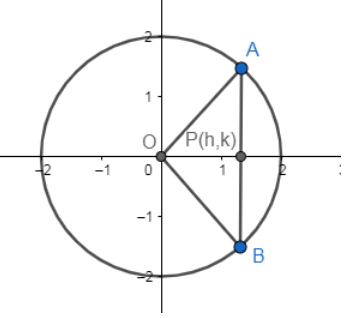Courses
Courses for Kids
Free study material
Offline Centres
MoreLast updated date: 06th Dec 2023
Total views: 381.6k
Views today: 8.81k

# The locus of the mid-points of the chord of the circle ${{x}^{2}}+{{y}^{2}}=4$ which subtends a right angle at the origin, is(a) $x+y=2$ (b) ${{x}^{2}}+{{y}^{2}}=1$(c) ${{x}^{2}}+{{y}^{2}}=2$(d) $x+y=1$Verified
381.6k+ views
Hint: Find the equation of chord with respect to the midpoint of chord which is given by the equation $T={{S}_{1}}$, T and ${{S}_{1}}$ are standard terms for conic sections chapter. Now homogenize the equation of the circle with the help of the equation of chord and use the condition given in the problem to get the locus.

Complete step-by-step answer: The given equation of circle is
${{x}^{2}}+{{y}^{2}}=4$…………….. (i)
Now, we need to determine the locus of the midpoint of the chord of the given circle if the chord is subtending a right angle at the origin.
Hence, the diagram can be given asWhere AB is the end points of the chord in the circle and $\angle AOB={{90}^{o}}$.
We can get center and radius of the given circle by comparing the given equation with the standard equation of circle i.e.,
${{x}^{2}}+{{y}^{2}}+2gx+2fy+c=0$
Where (-g,-f) is center and $\sqrt{{{g}^{2}}+{{f}^{2}}-c}$ is the length of the radius.
Hence, circle ${{x}^{2}}+{{y}^{2}}=4$ has center as (0, 0) and radius of 2 units.
We know that we can find equation of the chord with respect to midpoint of the chord by using the following reaction;
$T={{S}_{1}}$ ……….. (ii)
Where T can be given as
$x{{x}_{1}}+y{{y}_{1}}+2g\left( \dfrac{x+{{x}_{1}}}{2} \right)+2f\left( \dfrac{y+{{y}_{1}}}{2} \right)+c=0$ w.r.t point $\left( {{x}_{1}},{{y}_{1}} \right)$.
${{S}_{1}}$ can be given as,
${{S}_{1}}=x_{1}^{2}+y_{1}^{2}+2g{{x}_{1}}+2f{{y}_{1}}+c$ w.r.t point $\left( {{x}_{1}},{{y}_{1}} \right)$.
Hence, the equation of chord with respect to point (h, k) can be given using the relation $T={{S}_{1}}$ as
\begin{align} & hx+ky-4={{h}^{2}}+{{k}^{2}}-4 \\ & \Rightarrow hx+ky={{h}^{2}}+{{k}^{2}}..............(iii) \\ \end{align}
Now, we can get equation of pair of straight lines OA and OB by homogenizing equation of given circle with respect to the chord in following way:
From equation, we have
${{x}^{2}}+{{y}^{2}}=4\left( {{1}^{2}} \right)$ …………… (iv)
Now, we can rewrite the equation (iii) as
$\dfrac{hx+ky}{{{h}^{2}}+{{k}^{2}}}=1$ …………. (v)
And we can substitute ‘1’ in equation (iv) by using the above equation (v).
Hence, we get
${{x}^{2}}+{{y}^{2}}=4{{\left( \dfrac{hx+ky}{{{h}^{2}}+{{k}^{2}}} \right)}^{2}}$
Now, use ${{\left( a+b \right)}^{2}}={{a}^{2}}+{{b}^{2}}+2ab$ to simplify above equation. Hence, we get
\begin{align} & {{x}^{2}}+{{y}^{2}}=4\left( \dfrac{{{h}^{2}}{{x}^{2}}+{{k}^{2}}{{y}^{2}}+2hkxy}{{{\left( {{h}^{2}}+{{k}^{2}} \right)}^{2}}} \right) \\ & \Rightarrow \left( {{x}^{2}}+{{y}^{2}} \right){{\left( {{h}^{2}}+{{k}^{2}} \right)}^{2}}=4{{h}^{2}}{{x}^{2}}+4{{k}^{2}}{{y}^{2}}+8hkxy \\ & \Rightarrow {{x}^{2}}\left( {{\left( {{h}^{2}}+{{k}^{2}} \right)}^{2}}-4{{h}^{2}} \right)+{{y}^{2}}\left( {{\left( {{h}^{2}}+{{k}^{2}} \right)}^{2}}-4{{k}^{2}} \right)=8hkxy \\ & \Rightarrow {{x}^{2}}\left( {{\left( {{h}^{2}}+{{k}^{2}} \right)}^{2}}-4{{h}^{2}} \right)+{{y}^{2}}\left( {{\left( {{h}^{2}}+{{k}^{2}} \right)}^{2}}-4{{k}^{2}} \right)-8hkxy=0.........(vi) \\ \end{align}
Now, we get a pair of straight lines OA and OB by using the equation (vi). And we know they are perpendicular to each other as they are perpendicular to each other as they are subtending a right angle at origin.
Now, we know that standard equation of pair of straight lines passing through origin is $a{{x}^{2}}+b{{y}^{2}}+2hxy=0$ and lines will be perpendicular if
Coefficient of ${{x}^{2}}$+ coefficient of ${{y}^{2}}=0$
a + b = 0 …………. (vii)
Hence, we get from equation (vi) and (vii) as
\begin{align} & {{\left( {{h}^{2}}+{{k}^{2}} \right)}^{2}}-4{{h}^{2}}+{{\left( {{h}^{2}}+{{k}^{2}} \right)}^{2}}-4{{k}^{2}}=0 \\ & 2{{\left( {{h}^{2}}+{{k}^{2}} \right)}^{2}}-4\left( {{h}^{2}}+{{k}^{2}} \right)=0 \\ & 2\left( {{h}^{2}}+{{k}^{2}} \right)\left[ {{h}^{2}}+{{k}^{2}}-2 \right]=0 \\ \end{align}
Hence, we get equation from above relations as
${{h}^{2}}\text{+}{{k}^{2}}\text{=0, }{{h}^{2}}\text{+}{{k}^{\text{2}}}\text{=2}$
Now, we can replace (h, k) by (x, y) to get the required locus, hence, we get
${{x}^{2}}+{{y}^{2}}=0,{{x}^{2}}+{{y}^{2}}=2$
Now, ${{h}^{2}}+{{k}^{2}}=0$ is not possible for the given relations. Put ${{h}^{2}}+{{k}^{2}}=0$ to the equation (iii), we get equation of chord as
hx + ky = 0
Now, chord is passing through origin as per the above equation of the chord. And hence the chord will not subtend right angle $\left( {{90}^{o}} \right)$ at origin if it will pass through origin.
Hence, the only equation possible for getting locus would be
${{h}^{2}}+{{k}^{2}}=2$
Now, we can replace (h, k) by (x, y) to get the required locus, hence, we get
${{x}^{2}}+{{y}^{2}}=2$
So, option (c) is the correct answer.

Note: Another approach for the question would be that we can suppose parametric coordinates of point A and B as
$A\left( 2\cos {{\theta }_{1}},2\sin {{\theta }_{1}} \right),B\left( 2\cos {{\theta }_{2}},2\sin {{\theta }_{2}} \right)$
Now, find slopes of OA and OB and use relation ${{m}_{1}}{{m}_{2}}=-1$ for perpendicular lines. Now, put
\begin{align} & h=\dfrac{2\cos {{\theta }_{1}}+2\cos {{\theta }_{2}}}{2}=\cos {{\theta }_{1}}+\cos {{\theta }_{2}} \\ & k=\dfrac{2\sin {{\theta }_{1}}+2\sin {{\theta }_{2}}}{2}=\sin {{\theta }_{1}}+\sin {{\theta }_{2}} \\ \end{align}
And hence eliminates ${{\theta }_{1}},{{\theta }_{2}}$ to get the required locus.
Pair of homogenizing any curve f(x) w.r.t any given line cutting the curve and lines as well.
Homogenize f(x) w.r.t AC to get the equation of lines AB and BC together, where B should be origin.